# 2. Find r(t) if r'(t) = 5tại + vtj ifr(0) = 2i + j.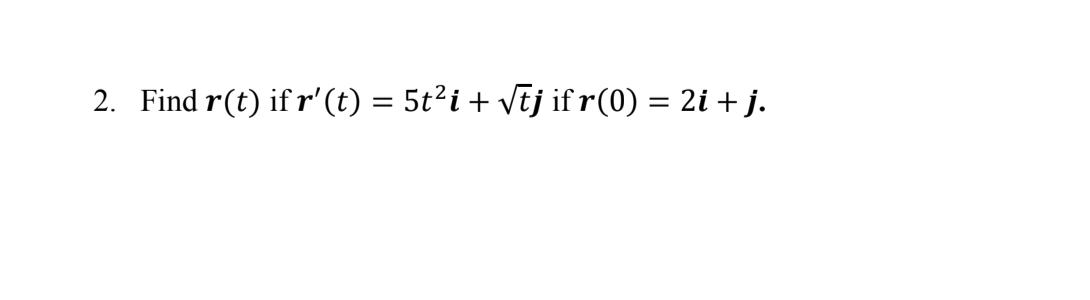2. Find r(t) if r'(t) = 5tại + vtj ifr(0) = 2i + j.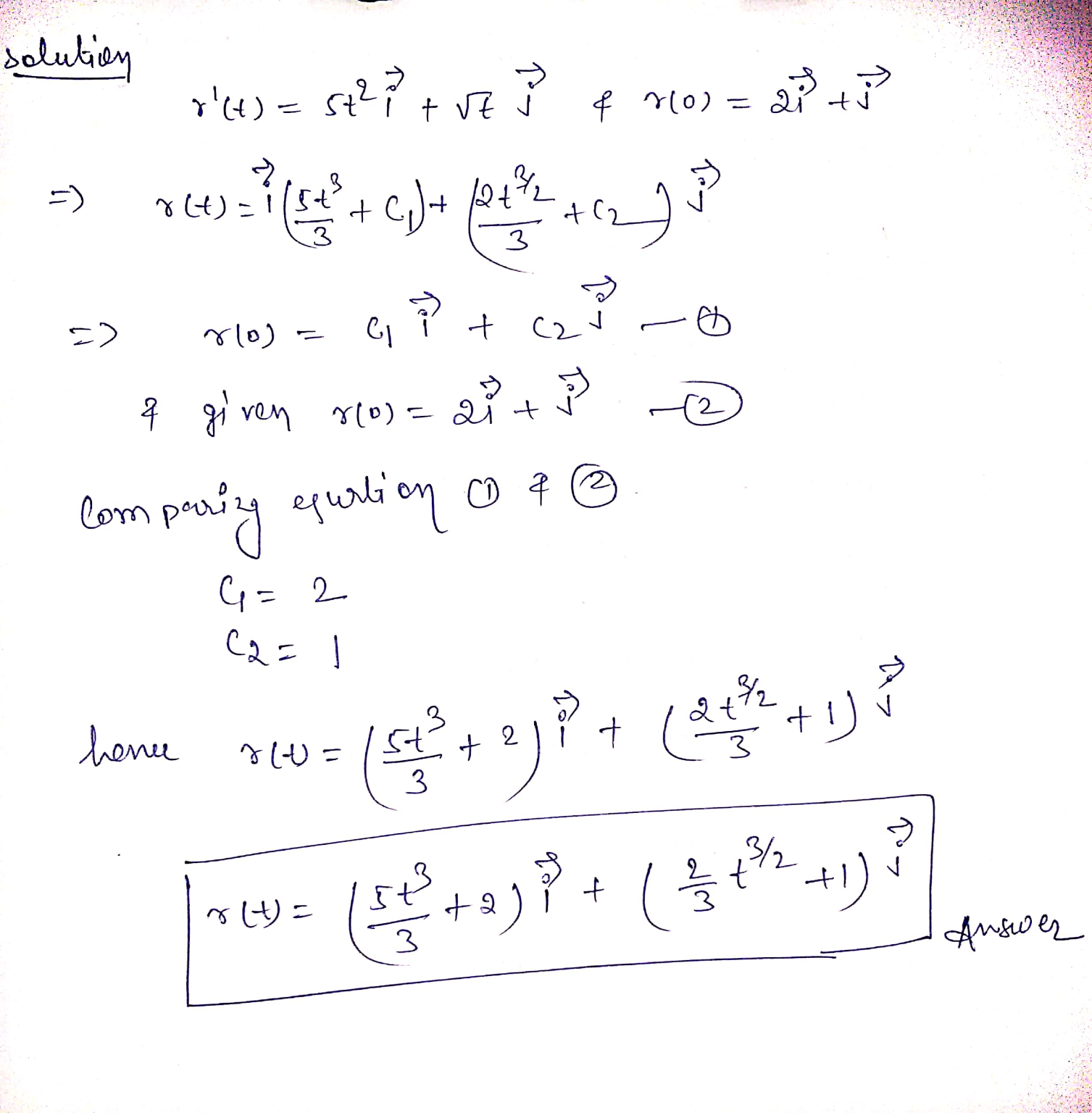##### Add Answer of: 2. Find r(t) if r'(t) = 5tại + vtj ifr(0) = 2i + j.
Similar Homework Help Questions
• ### 15) The acceleration at t 0 for r(t) - t2i + (8t3 - 2)j +N16-2tk 1...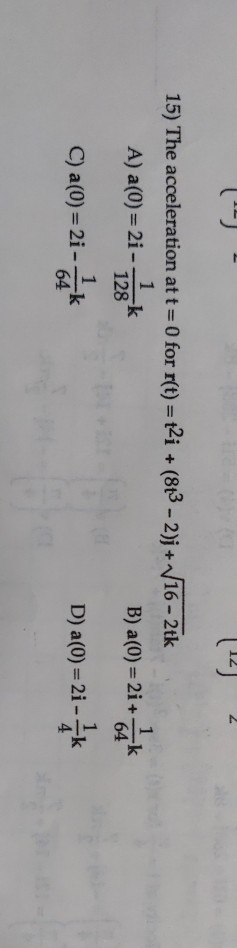15) The acceleration at t 0 for r(t) - t2i + (8t3 - 2)j +N16-2tk 1 A) a(0) 2i - 1 B) a(0)= 2i+ -k 64 k 128 1 C) a(0)= 2i- -21-1k k 64 D) a (0) 2i 4 15) The acceleration at t 0 for r(t) - t2i + (8t3 - 2)j +N16-2tk 1 A) a(0) 2i - 1 B) a(0)= 2i+ -k 64 k 128 1 C) a(0)= 2i- -21-1k k 64 D) a (0) 2i 4

• ### R programming Consider the continuous function r22r+3 ifr < 0 f(r)=r+3 12 +4 7 if 2...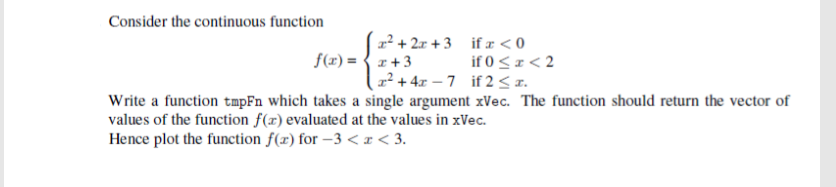R programming Consider the continuous function r22r+3 ifr < 0 f(r)=r+3 12 +4 7 if 2 < x. T>r50 Write a function tmpFn which takes a single argument xVec. The function should return the vector of values of the function f(x) evaluated at the values in xVec Hence plot the function f(x) for -3 <x< 3. Consider the continuous function r22r+3 ifr

• ### Find r(t)Find r(t) given that r''(t) = <, ,tan(t)>, r'(0) = i-2k, r(0)=2i-3j+k

• ### question about linear algebra 21. The following two lines := -i+j+ k + t(2i - 2j - 2k), t e R r and y2 1 = 2 -1 interse...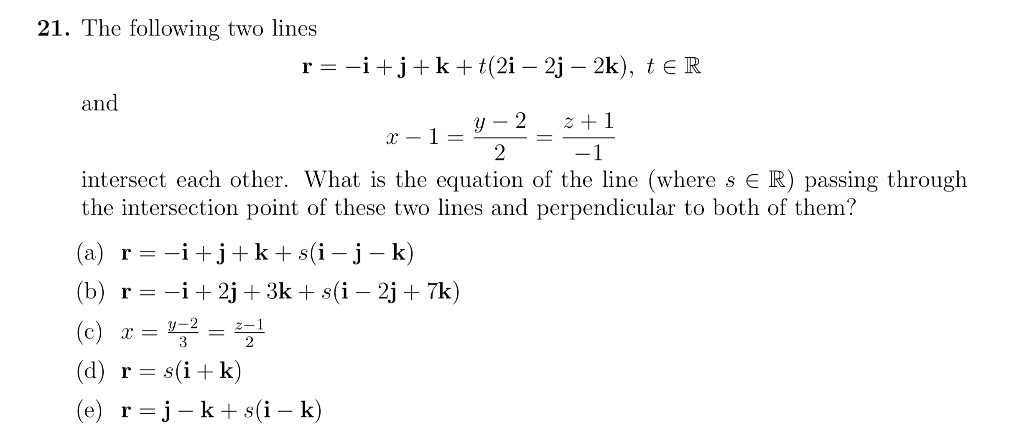question about linear algebra 21. The following two lines := -i+j+ k + t(2i - 2j - 2k), t e R r and y2 1 = 2 -1 intersect each other. What is the equation of the line (where s E R) passing through the intersection point of these two lines and perpendicular to both of them? r -ijk s(i - j - k) (a) (b) r i2j+3k + s(i - 2j + 7k) (c) (d) rsik) (e) =j-k s(i...

• ### Finding r(t)

Find r(t) ifr(0) = (0; 1;-1) andr'(t) = (e^3t; 2t sin t^2;2t -5)

• ### How to find r(t) if r'(t)=ti+e^tj+te^tk and r(0)=i+j+k?

#r'(t)=ti+e^tj+te^tk# #r(0)=i+j+k#

• ### Evaluate the line integral F(x,y)= (xy)i-(x^2)j, where C is given by r(t)=(t)i+(t^2)j for 0

Evaluate the line integral F(x,y)= (xy)i-(x^2)j, where C is given by r(t)=(t)i+(t^2)j for 0<t<2

• ### vectors - find r(t) #57

Find r(t)r'(t) = 2t j + √t kr(0) = i + jBook answer= i (t^2 + 1)j + (2/3)t^(3/2) k

• ### Vector Calc: graph r(t)Sketch the graph of r(t) and show the direction of increasingt.a.) r(t) = 2i + tjb.) r(t) = (1+cost)i + (3-sint)j ;c.) r(t) = coshti +sinhtjd.) r(t) = 2costi + 2sintj +tke.) r(t) = ti + (t^2)j+2k

• ### finding R(s) to find T(s) and N(s)

curve : r(t)= 3t^2i+2t^3j for (T is greater than or equal to 0)parametrize c by arc length parameter s (find r(s)), use r(s) to find t(s) and n(s)

Free Homework App